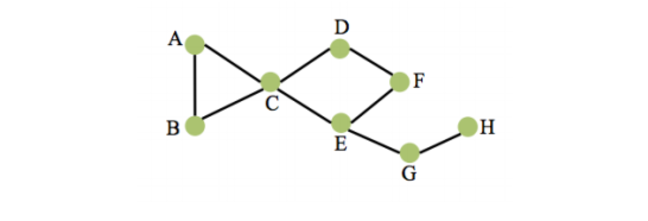## Want to keep learning?

This content is taken from the University of Southampton's online course, The Power of Social Media. Join the course to learn more.
1.14

# Local Clustering Co-efficient

## Essential knowledge:

• The Local Clustering Co-efficient tells us how connected the network is around a particular node. The clustering co-efficient is a fraction, representing the number of connections that exist as a proportion of the number that could exist.

• So for example, if I have three friends (Derek, Ed, and Freya), then those friends have three potential connections (Derek - Ed, Ed - Freya, Freya - Derek). If only one of those potential connections actually exists (for example if Derek is friends with Ed) then one out of three connections exist, giving me a local clustering co-efficient of 1/3.

## You may be interested that:

The local clustering co-efficient is a measure introduced by Watts and Strogatz in 1998 in their work to identify small world networks. It is calculated for each node in the network to examine the existing connections between its neighbouring nodes. In other words, it checks the existing connections between the neighbours of a given node to see whether they form a clique around that node.

Let’s look at the following example to illustrate the clustering co-efficient.Lets calculate the local clustering co-efficient for the node C:

• C’s neighbours are A, B, D and E.

• There is only one existing connection between C’s neighbours, and that is AB.

• There are six possible connections between its neighbours: AD, AE, BD, BE, DE and the one that is already there which is AB.

So the clustering co-efficient for C is 1/6

The clustering co-efficent is essentially a measure of how densely connected the network is around a particular node. So for example in a social network a person with a high clustering co-efficient is one whose friends tend to be friends with one another, forming a clique.

Thinking about your own social networks, are there ones in which your friends tend not to know one another, or ones in which they do (in other words where you have a particularly high or low clustering co-efficient)?